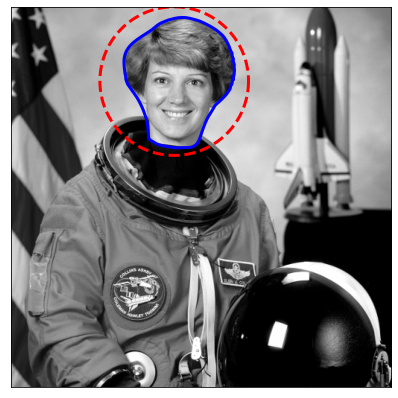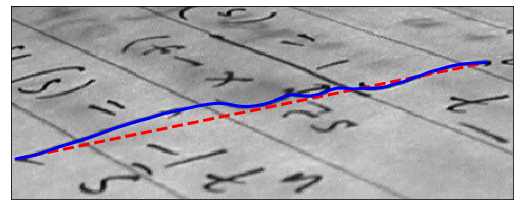# 2. Active contour model¶

The active contour model is a method to fit open or closed splines to lines or edges in an image. It works by minimizing an energy that is in part defined by the image and part by the spline’s shape: length and smoothness. The minimization is done implicitly in the shape energy and explicitly in the image energy.

In the following two examples, the active contour model is used

1. to segment the face of a person from the rest of an image by fitting a closed curve to the edges of the face and

2. to find the darkest curve between two fixed points while obeying smoothness considerations.

Typically it is a good idea to smooth images a bit before analyzing, as done in the following examples.

We initialize a circle around the astronaut’s face and use the default boundary condition bc='periodic' to fit a closed curve. The default parameters w_line=0, w_edge=1 will make the curve search towards edges, such as the boundaries of the face.

import numpy as np
import matplotlib.pyplot as plt
from skimage.color import rgb2gray
from skimage import data
from skimage.filters import gaussian
from skimage.segmentation import active_contour


## 2.1. Load the test image¶

img = data.astronaut()
img = rgb2gray(img)


## 2.2. Data for creating circular boundary¶

s = np.linspace(0, 2*np.pi, 400)
x = 220 + 100*np.cos(s)
y = 100 + 100*np.sin(s)
init = np.array([x, y]).T


## 2.3. Contour (after applying gaussian smoothing)¶

cntr = active_contour(gaussian(img, 3),init, alpha=0.015, beta=10, gamma=0.001)

c:\program files\python37\lib\site-packages\ipykernel_launcher.py:1: FutureWarning: The coordinates used by active_contour will change from xy coordinates (transposed from image dimensions) to rc coordinates in scikit-image 0.18. Set coordinates='rc' to silence this warning. coordinates='xy' will restore the old behavior until 0.18, but will stop working thereafter.
"""Entry point for launching an IPython kernel.

fig, ax = plt.subplots(figsize=(7, 7))
ax.imshow(img, cmap=plt.cm.gray)
# Circular boundary
ax.plot(init[:, 0], init[:, 1], '--r', lw=3)
# Detected contour
ax.plot(cntr[:, 0], cntr[:, 1], '-b', lw=3)
ax.set_xticks([]), ax.set_yticks([])
ax.axis([0, img.shape, img.shape, 0])

(0.0, 512.0, 512.0, 0.0)## 2.4. Text data image¶

Here we initialize a straight line between two points, (5, 136) and (424, 50), and require that the spline has its end points there by giving the boundary condition bc=’fixed’. We furthermore make the algorithm search for dark lines by giving a negative w_line value.

img = data.text()

x = np.linspace(5, 424, 100)
y = np.linspace(136, 50, 100)
init = np.array([x, y]).T

cntr = active_contour(gaussian(img, 1), init, bc='fixed',
alpha=0.1, beta=1.0, w_line=-5, w_edge=0, gamma=0.1)

fig, ax = plt.subplots(figsize=(9, 5))
ax.imshow(img, cmap=plt.cm.gray)
ax.plot(init[:, 0], init[:, 1], '--r', lw=3)
ax.plot(cntr[:, 0], cntr[:, 1], '-b', lw=3)
ax.set_xticks([]), ax.set_yticks([])
ax.axis([0, img.shape, img.shape, 0])

plt.show()

c:\program files\python37\lib\site-packages\ipykernel_launcher.py:6: UserWarning: The keyword argument bc to active_contour has been renamed. Use boundary_condition= instead. bc will be removed in scikit-image v0.18.

c:\program files\python37\lib\site-packages\ipykernel_launcher.py:6: FutureWarning: The coordinates used by active_contour will change from xy coordinates (transposed from image dimensions) to rc coordinates in scikit-image 0.18. Set coordinates='rc' to silence this warning. coordinates='xy' will restore the old behavior until 0.18, but will stop working thereafter.